# JEE Main 2019 April Chemistry Paper with Solutions

IIT JEE aspirants can refer to this page to find the answers for all the Chemistry questions asked in JEE 2019 April. These questions are solved by our subject experts. One of the best ways to prepare for the examination is by practising previous year question papers. Practise these questions to get an excellent score in the examination.

### April Paper - Chemistry

1. Which of the following amine will be prepared by Gabriel phthalimide reaction?

1. a. n-Butylamine
2. b. Triethylamine
3. c. neo-Pentylamine
4. d. tert-Butylamine

Solution:

1. Gabriel pthalimide synthesis is used to prepare 10 amines only

2. Reaction of dilute HCl with Maltose gives:

1. a. D – glucose
2. b. D – fructose
3. c. D – glucose and D – fructose
4. d. D – galactose

Solution:

1. Maltose is a dissacalaride, consisting of 2 glucose units. So on hydrolysis, it gives only D-glucose.

3. The correct order of Kb value of the following is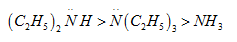1. a. 1 > 2 > 3
2. b. 1 > 3 > 2
3. c. 3 > 2 > 1
4. d. 3 > 1 > 2

Solution:

1. kb ∝ Basic strength.

Basic strength ∝ amount of hydration ∝ Availability of Lone Pairs on Nitrogen.

Combining both effects, the order of Basic strength is, 20> 30> NH3

4. Write IUPAC name of the following compound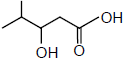1. a. 3-Hydroxy-2-methylpentanoic acid
2. b. 4-Methyl-3-hydroxypentanoic acid
3. c. 3-Hydroxy-4-methylpentanoic acid
4. d. 2-Methyl-2-hydroxypentanoic acid

Solution:

1.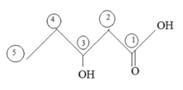3-hydroxy 4-methyl Pentanoic acid

5. Compound ‘X’ will be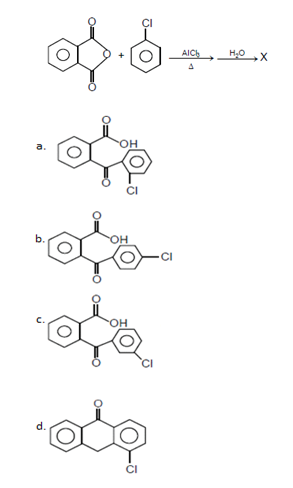Solution:

1.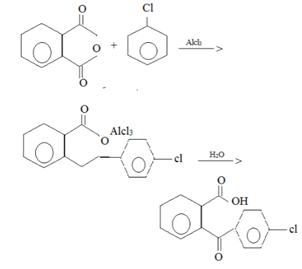6. X will be: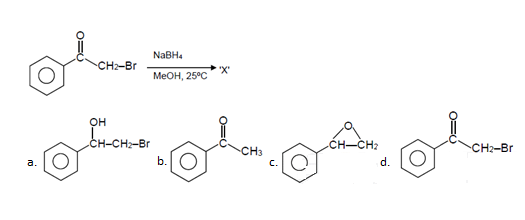Solution:

1.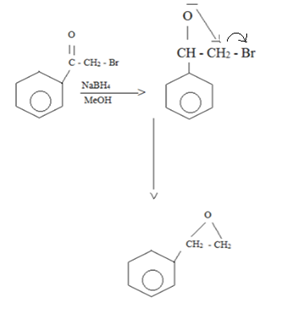7.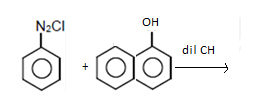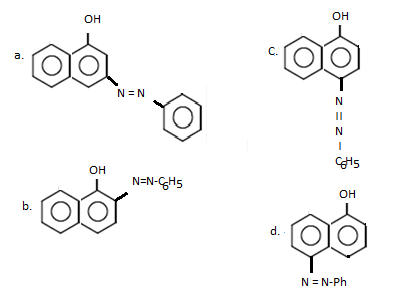Solution:

1. In a2o coupling reaction, the Hydrogen is taken from the less hindered para position.

8.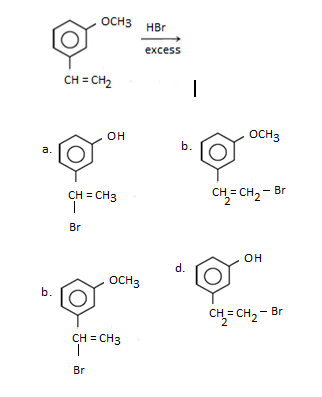Solution:

1.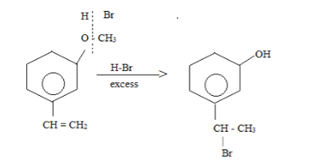Two reaction occur simultaneously

a) Ether hydrolysis using HBr

9. Find the compound ‘X’ which give following test.

Neutral FeCl3 -ve

Fehling solution -ve

Iodoform reaction +ve

Grignard reagent +ve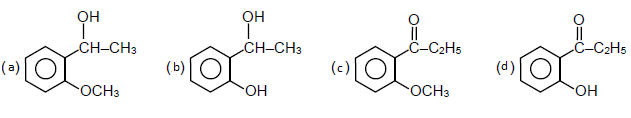Solution:

1.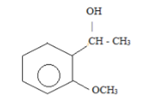Neutre Fecl3 → - ve (phenol is absent)

Fehling test → - ve (no CHO)

Iodoform → + ve (α – Methyl group is present)

Grignard → acidic H-atom is present

10. Identify the compound ‘X’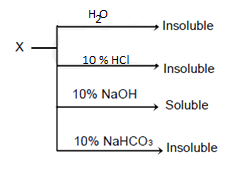1. a. Toluidine
2. b. Benzamide
3. c. Para-Cresol
4. d. Oleic acid

Solution:

1. Para-cresol is acidic enough to be soluble in 10% NaOH. But Oleic acid forms salt, but is insoluble due to presence & long chain of Hydrocarbon

11. Which of the following is not correct for an ideal gas as per first low of thermodynamics

1. a. Adiabatic ΔU = -w
2. b. Isothermal q = -w
3. c. cyclic q = -w
4. d. Isochoric ΔU = q

Solution:

1. From 1st Law,

∆U = q + ω

Therefore,∆U = ω

12. In Freundlich isotherm,

$$\frac{x}{m}\propto {{P}^{a}}.$$
Find the value of a from the following graph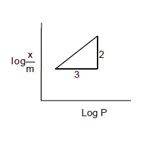1. a.
$$\frac{2}{3}$$
2. b.
$$\frac{1}{3}$$
3. c.
$$\frac{3}{2}$$
4. d. 1

Solution:

1. Freundlich Isotherm equations:

$$\log \frac{x}{m}=\log k+\frac{1}{n}\log P$$

$$y=c+mx$$

$$m=\frac{1}{n}=\frac{2}{3}.$$

13. In a mixture of A and B having vapour pressure of pure A and pure B are 400 mm Hg and 600 mm Hg respectively, mole fraction of B in liquid phase is 0.5. Calculate total vapour pressure and mole fraction of A and B in vapour phase

1. a.500, 0.4, 0.6
2. b.500, 0.5, 0.5
3. c.450, 0.4, 0.6
4. d.450, 0.5, 0.5

Solution:

1. $${{P}_{T}}=P\,_{A\times A}^{o}+P\,_{B\times B}^{o}$$

= 0. 5 × 400 + 0.5 × 600

= 500 mm of Hg

$$\frac{1}{{{P}_{T}}}=\frac{{{Y}_{A}}}{400}+\frac{1-{{Y}_{B}}}{600}$$

$$∴ {{Y}_{A}}=0.6$$

$${{Y}_{B}}=1-0.6=0.4$$

14. Arrange the following set of quantum numbers having highest energy of an electron.

(p) n = 4 l = 1 m = +1

$$s = +\frac{1}{2}$$

(q) n = 4 l = 2 m = -1 s

$$=-\frac{1}{2}$$

(r) n = 3 l = 2 m = 0 s

$$=+\frac{1}{2}$$

(s) n = 3 l = 1 m + 1 s

$$=-\frac{1}{2}$$

1. a. q > r > p > s
2. b. q > p > r > s
3. c. s > p > r > q
4. d. s > r > p > q

Solution:

1. We know that n is principle quantum number and l is an azimuthal quantum number.

Also, l=n−1

The set of quantum number which have highest value of n+l will have the highest energy and vice versa.

$$E\,\alpha \,\left( n+l \right)$$

15. A forms ccp lattice, B occupy half of the octahedral voids and ‘O’ occupy all the tetrahedral voids. Calculate formula –

1. a. A2BO4
2. b. ABO4
3. c. A2B2O
4. d. A2B2O

Solution:

1. CCP lattice of A → no of atom of A per unit cell → 4.

N oct. voids = 2 N tetra.Voids. But B occupies only half of oct. voids

&therefore; No of atoms of B =

$$4\times \frac{1}{2}=2$$

No of atoms of C → 4 × 2 = 8

&therefore; The formula → A4 B2 C8

16. B2H6 reacts with O2 and H2O respectively to form

1. a. B2O3, H3BO3
2. b.
$${{B}_{2}}{{O}_{3}},B{{H}_{{{4}^{-}}}}$$
3. c. HBO2, H3BO3
4. d. H3BO3, HBO2

Solution:

1. B2H6 reacts with O2 and H2O to form Diborane extensively burns in oxygen to form basic oxide and B2H6 hydrolysis to give Boric acid

$${{B}_{2}}{{H}_{6}}+3{{O}_{2}}\to {{B}_{2}}{{O}_{3}}+3{{H}_{2}}O$$

$${{B}_{2}}{{H}_{6}}+6{{H}_{2}}O\to 2{{H}_{3}}B{{O}_{3}}+3{{H}_{2}}$$

17. Solution of 100 ml water contains 0.73 g of Mg(HCO3)2 and 0.81 g of Ca(HCO3)2. Calculate the hardness in terms of ppm of CaCO3.

1. a. 102 ppm
2. b. 104 ppm
3. c. 5 × 103 ppm
4. d. 103 ppm

Solution:

1. Number of moles of

$$Mg{{\left( HC{{O}_{3}} \right)}_{2}}=\frac{0.73}{146}=0.005$$

Number of moles of

$$Ca{{\left( HC{{O}_{3}} \right)}_{2}}=\frac{0.81}{162}=0.005$$

$$PPM=\frac{Total\,no.of\,mole\times 100\times {{10}^{6}}}{100}$$

$$=\frac{0.005+0.005\times 100\times {{10}^{6}}}{100}$$

$$={{10}^{4}}PPM$$
.

18. For

$$Z{{r}_{3}}{{(P{{O}_{4}})}_{4}}$$
the solubility product is Ksp and solubility is S. Find the correct relation.

1. a.
$$S={{\left( \frac{{{K}_{sp}}}{6912} \right)}^{1/7}}$$
2. b.
$$S={{\left( \frac{{{K}_{sp}}}{216} \right)}^{1/7}}$$
3. c.
$$S={{\left( \frac{{{K}_{sp}}}{216} \right)}^{1/8}}$$
4. d.
$$S={{\left( \frac{{{K}_{sp}}}{912} \right)}^{1/3}}$$

Solution:

1. It is A3B4 type of salt

Hence

$$K{{s}_{p}}={{\left( 3s \right)}^{3}}\times {{\left( 4s \right)}^{4}}$$

$$=27{{s}^{3}}\times 256{{s}^{4}}$$

$$K{{s}_{p}}=6912{{s}^{7}}$$

$$S=\sqrt{\frac{K{{s}_{P}}}{6912}}$$

19. Given complexes are low spin complexes,

$${{[V{{(CN)}_{6}}]}^{4-}}$$

$${{[Cr{{(N{{H}_{3}})}_{6}}]}^{2+}}$$

$${{[Ru{{(N{{H}_{3}})}_{6}}]}^{3+}}$$

$${{[Fe{{(CN)}_{6}}]}^{4-}}$$

Then order of magnetic moment (μ) for

$${{V}^{2+}},F{{e}^{2+}},C{{r}^{+3}},R{{u}^{3+}}$$
is

1. a.
$${{V}^{2+}}>C{{r}^{2+}}>F{{e}^{2+}}>R{{u}^{3+}}$$
2. b.
$$F{{e}^{2+}}>{{V}^{2+}}>C{{r}^{+2}}>R{{u}^{3+}}$$
3. c.
$${{V}^{2+}}>C{{r}^{2+}}>R{{u}^{3+}}>F{{e}^{2+}}$$
4. d.
$$F{{e}^{2+}}>C{{r}^{3+}}>{{V}^{2+}}>R{{u}^{3+}}$$

Solution:

1. For

$${{\left[ V{{\left( CN \right)}_{6}} \right]}^{-4}}$$

Magnetic moment

$$=\sqrt{3\left( 3+2 \right)}=\sqrt{15}BM=3.9BM$$

$${{\left[ Cl{{\left( N{{H}_{3}} \right)}_{6}} \right]}^{2+}}$$

Magnetic moment

$$=\sqrt{2\left( 2+2 \right)}=\sqrt{8}=2.9BM$$

$${{\left[ Ru{{\left( N{{H}_{3}} \right)}_{6}} \right]}^{+3}}$$

Magnetic moment

$$=\sqrt{1\left( 1+2 \right)}=\sqrt{3}=1.732$$

$${{\left[ Fe{{\left( CN \right)}_{6}} \right]}^{-4}}$$

Magnetic moment =

$$\sqrt{no\,\,unpaired}=0$$

Hence order

$${{V}^{+2}}>C{{l}^{+2}}R{{u}^{+3}}>F{{e}^{+2}}$$

20. Given

$$E{{{}^\circ }_{{{S}_{2}}O_{8}^{2-}/SO_{4}^{2-}}}=2.05V$$

$$E_{B{{r}_{2}}/B{{r}^{-}}}^{o}=1.40V$$

$$E_{A{{u}^{3+}}/Au}^{o}=1.10V$$

$$E_{{{O}_{2}}/{{H}_{2}}O}^{o}=1.20V$$

Which of the following is the strongest oxidizing agent?

1. a.
$${{S}_{2}}O_{8}^{2-}$$
2. b.Br2
3. c.Au+3
4. d.O2

Solution:

1. More is Positive standard reduction Potential more is oxidizing nature. hence

$${{S}_{2}}O_{8}^{-2}$$

21. Assertion: Ozone is getting depleted due to CFCs
Reason: With the deplection of ozone layer more UV radiation filters into troposphere

1. a. Both Assertion and Reason are true and Reason is the correct explanation of Assertion.
2. b. Both Assertion and Reason are true but Reason is not correct explanation of Assertion.
3. c. Assertion is true but Reason is false.
4. d. Assertion is false but Reason is true.

Solution:

1. Ozone gets decomposed by CFCS hence Assertion is correct

Ozone depletion cause, harmful uv rays to enter troposphere hence reason is correct but reason is not correct explanation of assertion, 

22. In which of the following order of hydration energy correct.

1. a.
$$L{{i}^{+}}>N{{a}^{+}}>{{K}^{+}}>R{{b}^{+}}>C{{s}^{+}}$$
2. b.
$$L{{i}^{+}}<N{{a}^{+}}<{{K}^{+}}<R{{b}^{+}}<C{{s}^{+}}$$
3. c.
$$L{{i}^{+}}>N{{a}^{+}}>{{K}^{+}}>C{{s}^{+}}>R{{b}^{+}}$$
4. d.
$$L{{i}^{+}}<N{{a}^{+}}<{{K}^{+}}<C{{s}^{+}}<R{{b}^{+}}$$

Solution:

1. Hydration energy α charge, αsize1

$$L{{i}^{+}}>N{{a}^{+}}>{{K}^{+}}>R{{b}^{+}}>C{{s}^{+}}$$

23. Ellingham diagram is used for

1. a.Reduction
2. b.Electrolysis
3. c.Zone refining
4. d.Van-Arkel

Solution:

1. It is used for estimating, the temperature at which a particular metal oxide is reduced by another metal.

24. In isoelectronic species

$$C{{l}^{-}},Ar,C{{a}^{2+}}$$
size differ due to

1. a.Nuclear charge
2. b.Electronic – electronic repulsion in valence shell
3. c.Magnetic quantum number
4. d.Principal quantum number

Solution:

1. In isoelectronic species, number of electrons are same but size decrease with increase in atomic number or nuclear charge.

25. Three mole of Ag is heated from 300 K to 1000 K. Calculate ΔH when P = 1 atm and CP = 23 + 0.01 T.

1. a. 62 kJ/mol
2. b. 45 kJ/mol
3. c. 38 kJ/mol
4. d. 54 kJ/mol

Solution:

1. $$\Delta H=\int\limits_{{{T}_{1}}}^{{{T}_{2}}}{n\,CpdT}$$

$$n\int\limits_{30}^{400}{\left( 23+0.1T \right)dT}$$

$$=3\left[ 23T+\frac{{{0.17}^{2}}}{2} \right]_{300}^{400}$$

$$=61960J/mol\Rightarrow 62k{}^\circ mol$$

26.

$$Fe{{C}_{2}}{{O}_{4}},F{{e}_{2}}{{({{C}_{2}}{{O}_{4}})}_{3}},FeS{{O}_{4}},F{{e}_{2}}{{(S{{O}_{4}})}_{3}}$$
one mole each, will react with how many moles of
$$KMn{{O}_{4}}$$

1. a.1
2. b.2
3. c.3
4. d.5

Solution:

1. $$Fe{{C}_{2}}{{O}_{4}}+KMn{{O}_{4}}$$
$${{}}F{{e}^{3+}}+C{{o}_{2}}+M{{n}^{2+}}$$

$$F{{e}_{2}}{{\left( {{C}_{2}}{{O}_{4}} \right)}_{3}}+MnO_{4}^{-}$$
$${{}}F{{e}^{3+}}$$

$$F{{e}_{3}}{{O}_{4}}$$
$${{}}F{{e}^{3+}}$$

∴ Total:

$$\frac{3}{3}+\frac{6}{5}+\frac{1}{5}=2$$

27. For 2A + B -> C. Find the rate low.

 [A] [B] Initial Rate 0.05 0.05 0.045 0.10 0.05 0.09 0.20 0.10 0.72

1. a. R = K[A] [B]
2. b. R = k[A] [B]2
3. c. R = k[A2][B]
4. d. R = k[A]2 [B]2

Solution:

1. $${{v}_{1}}=K{{\left[ {{A}_{1}} \right]}^{x}}{{\left[ B \right]}^{4}}$$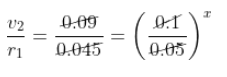$$2={{2}^{x}}$$

$$x=1$$
.

$$\frac{0.79}{0.09}={{\left( \frac{0.2}{0.1} \right)}^{1}}{{\left[ \frac{0.1}{0.05} \right]}^{4}}$$

$$8={{2}^{1}}{{2}^{y}}$$

$${{2}^{y}}=4$$

$$y=2$$

28. Which of the following lanthanoid ions are coloured?

1.Lu+3

2. Pm+3

3.Sm+3

4.Eu+3

1. a.
$$L{{u}^{+3}},P{{m}^{+3}},S{{m}^{+3}}$$
2. b.
$$P{{m}^{+3}},S{{m}^{+3}},E{{u}^{+3}}$$
3. c.
$$L{{u}^{+3}},P{{m}^{+3}},S{{n}^{+3}}$$
4. d.
$$L{{u}^{+3}},S{{m}^{+3}},E{{u}^{+3}}$$

Solution:

1. $$P{{m}^{+3}},S{{m}^{+7}},E{{u}^{+7}}$$
contain unpaired
$$f-$$
electrons. Hence coloured.

### JEE Main 2019 April Chemistry Paper with Solutions